Back to Adaptive Vision Studio website

You are here: Start » Filter Reference » Integer

Integer

Select a filter from the list below.

IconNameDescription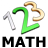AbsoluteIntegerComputes the absolute value of a number.AddIntegersComputes the sum of numbers.AddIntegers_OfArrayComputes the sum of numbers of an array.AddIntegers_OfLoopComputes the sum of numbers appearing in consecutive iterations.AddLongIntegersComputes the sum of Long numbers.AddLongIntegers_OfArrayComputes the sum of Long numbers of an array.AddLongIntegers_OfLoopComputes the sum of Long numbers appearing in consecutive iterations.AverageIntegersReturns the average of two numbers.AverageIntegers_OfArrayReturns the average value of integers.AverageIntegers_OfArray_OrNilReturns the average value of integers; returns NIL if the array is empty.AverageIntegers_OfLoopReturns the average of the numbers appearing in consecutive iterations.AverageLongIntegersReturns the average of two Long numbers.AverageLongIntegers_OfArrayReturns the average value of Long integers.AverageLongIntegers_OfArray_OrNilReturns the average value of Long integers; returns NIL if the array is empty.AverageLongIntegers_OfLoopReturns the average of the Long numbers appearing in consecutive iterations.ConvertBinaryDigitsToIntegerConverts binary digits to integer using least significant bit order.ConvertIntegerToBinaryDigitsConverts integer to binary digits using least significant bit order.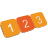CreateIntegerSequenceCreates an arithmetic sequence of integer numbers.DivideIntegersComputes the quotient of two numbers.DivideLongIntegersComputes the quotient of two numbers.IntegerDifferenceComputes the absolute value of two numbers difference.LerpIntegersLinearly interpolates between two integers.LongDifferenceComputes the absolute value of two Long numbers differenceMaximumIntegerReturns the higher of two numbers.MaximumInteger_OfArrayReturns the greatest value in an array of integer numbers.MaximumInteger_OfArray_OrNilReturns the greatest value in an array of integer numbers; returns NIL if the array is empty.MaximumInteger_OfLoopReturns the greatest of numbers appearing in consecutive iterations.MaximumLongIntegersReturns the higher of two Long Integer numbers.MaximumLongIntegers_OfArrayReturns the greatest value in an array of Long Integer numbers.MaximumLongIntegers_OfArray_OrNilReturns the greatest value in an array of Long Integer numbers; returns NIL if the array is empty.MaximumLongIntegers_OfLoopReturns the greatest of Long Integer numbers appearing in consecutive iterations.MinimumIntegerReturns the lower of two numbers.MinimumInteger_OfArrayReturns the smallest value in an array of Long Integer numbers.MinimumInteger_OfArray_OrNilReturns the smallest value in an array of Long Integer numbers; returns NIL if the array is empty.MinimumInteger_OfLoopReturns the smallest of numbers appearing in consecutive iterations.MinimumLongIntegersReturns the lower of two Long Integer numbers.MinimumLongIntegers_OfArrayReturns the smallest value in an array of Long Integer numbers.MinimumLongIntegers_OfArray_OrNilReturns the smallest value in an array of Long Integer numbers; returns NIL if the array is empty.MinimumLongIntegers_OfLoopReturns the smallest of Long Integer numbers appearing in consecutive iterations.ModuloComputes remainder of integer division of two numbers.MultiplyIntegersComputes the product of numbers.MultiplyIntegers_OfArrayComputes the product of numbers of an array.MultiplyIntegers_OfLoopComputes the product of numbers appearing in consecutive iterations.MultiplyLongIntegersComputes the product of long numbers.MultiplyLongIntegers_OfArrayComputes the product of long numbers of an array.MultiplyLongIntegers_OfLoopComputes the product of numbers appearing in consecutive iterations.NegateIntegerComputes the negation of a number.ParseIntegerReturns the integer number represented by a string.ParseLongIntegersReturns the long integer number represented by a string.RescaleIntegerApplies linear transformation to integer.SubtractIntegersComputes the difference of two numbers.SubtractLongIntegersComputes the difference of two Long numbers.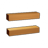TestIntegerEqualToChecks whether two integers are equal.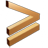TestIntegerGreaterOrEqualCompares two integers with the >= operator.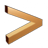TestIntegerGreaterThanCompares two integers with the > operator.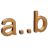TestIntegerInRangeChecks whether an integers is in the specified range.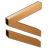TestIntegerLessOrEqualCompares two integers with the <= operator.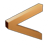TestIntegerLessThanCompares two integers with the < operator.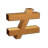TestIntegerUnequalToChecks whether two integers are unequal.TestLongIntegerEqualToChecks whether two Long integers are equal.TestLongIntegerGreaterOrEqualCompares two Long integers with the >= operator.TestLongIntegerGreaterThanCompares two Long integers with the > operator.TestLongIntegerInRangeChecks whether Long integers is in the specified range.TestLongIntegerLessOrEqualCompares two Long integers with the <= operator.TestLongIntegerLessThanCompares two Long integers with the < operator.TestLongIntegerUnequalToChecks whether two Long integers are unequal.